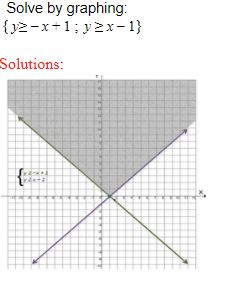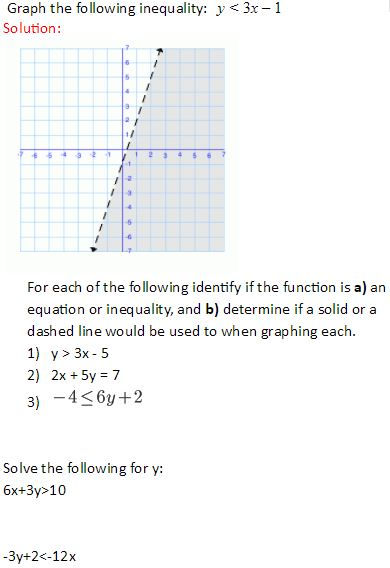#### MAT-HS.A-REI.12

 MAT-HS Targeted Standards(A) Concept: Algebra(REI) Domain: Reasoning with Equations and InequalitiesCluster: Represent and solve equations and inequalities graphically MAT-HS.A-REI.12 Graph the solutions to a linear inequality in two variables as a half-plane. Graph the solution set to a system of linear inequalities in two variables as the intersection of the corresponding half-planes.

## Student Learning Targets:

• I can
• I can

• I can
• I can

### Skills (Performance) Targets

• I can graph the solutions to a linear inequality in two variables, including dashed or solid boundary lines when appropriate.
• I can graph the solution set to a system of linear inequalities in two variables as their corresponding intersection.

• I can
• I can

## Proficiency Scale

 Score Description Sample Activity 4.0(advanced) In addition to achieving level 3.0 content, the student makes in-depth inferences and applications that go beyond what was taught3.5 In addition to Score 3.0 performance, the student demonstrates in-depth inferences and applications regarding the more complex content with partial success. 3.0(proficient) The student can: graph the solutions to a linear inequality in two variables, including dashed or solid boundary lines when appropriate. graph the solution set to a system of linear inequalities in two variables as their corresponding intersection. The student exhibits no major errors or omissions.2.5 The student demonstrates no major errors or omissions regarding the simpler details and processes (Score 2.0 content) and partial knowledge of the more complex ideas and processes (Score 3.0 content). 2.0(progressing) There are no major errors or omissions regarding the simpler details and processes as the student can: recognize and recall basic vocabulary, such as: linear inequality. linear equation. understand when to use a solid line vs a dashed line. understand that there is a region of solutions and how to identify it. graph a linear equation as the boundary line. solve for Y in a linear equation or inequality. However, the student exhibits major errors or omissions regarding the more complex ideas and processes.1.5 The student demonstrates partial knowledge of the simpler details and processes (Score 2.0 content) but exhibits major errors or omissions regarding the more complex ideas and procedures (Score 3.0 content). 1.0(beginning) With help, a partial understanding of some of the simpler details and processes and some of the more complex ideas and processes. - 0.5 With help, the student demonstrates a partial understanding of some of the simpler details and processes (Score 2.0 content) but not the more complex ideas and processes (Score 3.0 content).

## Resources

 Web Vocab half-plane